# Recursive version of integration by parts

## General description of technique

The general procedure is as follows:

1. Start doing the integration by parts.
2. If necessary, apply integration by parts twice OR use some trigonometric identity with the ultimate goal of seeing the original integration appear again. Note that you should take care to avoid the circular trap.
3. Denote by$I$ the particular choice of antiderivative for which the left and right sides are equal on the nose,i.e., not just up to additive constants.
4. Solve the linear equation in$I$.
5. The general indefinite integral is this solution plus an arbitrary additive constant.

## Examples

### Sine-squared function

For further information, refer: Sine-squared function#Integration

There are many ways of integrating$\sin^2$. One of these uses the recursive version of integration by parts. This method is given below:$\int \sin^2x \, dx = (\sin x)(-\cos x) - \int (\cos x)(-\cos x) \, dx = -\sin x \cos x + \int \cos^2 x \, dx$

We now rewrite$\cos^2x = 1 - \sin^2x$ and obtain: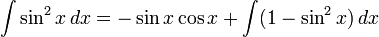$\int \sin^2x \, dx = -\sin x \cos x + \int (1 - \sin^2x) \, dx$

Setting$I$ to be a choice of antiderivative so that the above holds without any freely floating constants, we get:$\! I = -\sin x \cos x + x - I$

Rearranging, we get: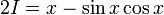$\! 2I = x - \sin x \cos x$

This gives:$I = \frac{x - \sin x \cos x}{2}$

So the general antiderivative is: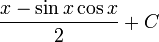$\frac{x - \sin x \cos x}{2} + C$

### Secant-cubed function

For further information, refer: Secant-cubed function#Integration

We rewrite$\sec^3x = \sec x \cdot \sec^2x$ and perform integration by parts, taking$\sec^2$ as the part to integrate. We use that an antiderivative of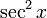$\sec^2 x$ is$\tan x$ whereas the derivative of$\sec x$ is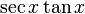$\sec x \tan x$: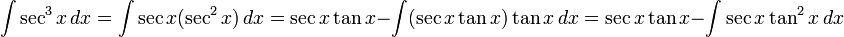$\int \sec^3 x \, dx = \int \sec x (\sec^2 x) \,dx = \sec x \tan x - \int (\sec x \tan x) \tan x \, dx = \sec x \tan x - \int \sec x \tan^2x \, dx$

We now use the fact that$\tan^2 x + 1 = \sec^2 x$, or more explicitly,$\tan^2 x = \sec^2 x - 1$, to rewrite this as:$\! \int \sec^3 x \, dx = \sec x \tan x - \int \sec x (\sec^2 x - 1) \, dx = \sec x \tan x x - \int \sec^3 x \, dx + \int \sec x \, d$

We now use the integration of the secant function to simplify this as: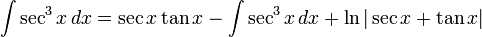$\! \int \sec^3 x \, dx = \sec x \tan x - \int \sec^3 x \, dx + \ln|\sec x + \tan x|$

We can choose an antiderivative$I$ of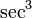$\sec^3$ so that the above equality (between the left-most and right-most expression) holds without any additive constant adjustment, and we get: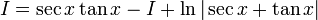$\! I = \sec x \tan x - I + \ln|\sec x + \tan x|$

We rearrange and obtain:$2I = \sec x \tan x + \ln|\sec x + \tan x|$

Dividing by 2, we get:$\! I = \frac{\sec x \tan x + \ln|\sec x + \tan x|}{2}$

The general antiderivative expression is thus: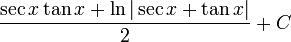$\frac{\sec x \tan x + \ln|\sec x + \tan x|}{2} + C$

### Exponential times cosine function

Consider the integration problem: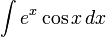$\int e^x \cos x \, dx$

We proceed by taking$e^x$ as the part to integrate and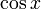$\cos x$ as the part to differentiate. We get: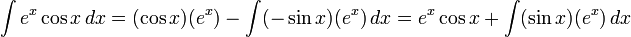$\int e^x \cos x \, dx = (\cos x)(e^x) - \int (-\sin x)(e^x) \, dx = e^x \cos x + \int (\sin x)(e^x) \, dx$

To avoid the circular trap, we should pick the exponential function as the part to integrate again, getting:$\int e^x \cos x \, dx = (\cos x)(e^x) + (\sin x)(e^x) - \int (\cos x)(e^x) \, dx$

Now, we take$I$ as the antiderivative where the above holds without additive constants, and get:$I = e^x(\cos x + \sin x) - I$

Rearranging, we get: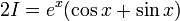$2I = e^x(\cos x + \sin x)$

This gives us: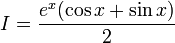$I = \frac{e^x(\cos x + \sin x)}{2}$

So the general integral is: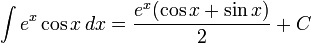$\int e^x \cos x \, dx = \frac{e^x(\cos x + \sin x)}{2} + C$